# Important DI Table for SBI PO Mains – 75

Directions (1-5): A school has four sections A, B, C, D of Class IX students.

The results of half yearly and annual examinations are shown in the table given below.

 Result No. of Students Sec- A Sec B Sec C Sec D Students failed in both Exams 28 23 17- 27 Students failed in half-yearly but passed in Annual Exams 14 12 8 13 Students passed in half-yearly but failed in Annual Exams 6 17 9 15 Students passed in both Exams 64 55 46 76

1. If the number of students passing an examination be considered a criteria for comparisons of difficulty level of two examinations, which of the following statements is true in this context?

(a) Half yearly examinations were more difficult.

(b) Annual examinations were more difficult.

(c) Both the examinations had almost the same difficulty level.

(d) The two examinations cannot be compared for difficulty level.

(e) None of these

(c) Both the examinations had almost the same difficulty level.

Number of students who passed half-yearly exams in the school

= (Number of students passed in half-yearly but failed in annual exams)

+ (Number of students passed in both exams)

= (6 + 17 + 9 + 15) + (64 + 55 + 46 + 76)

= 288.

Also, Number of students who passed annual exams in the school

= (Number of students failed in half-yearly but passed in annual exams)

+ (Number of students passed in both exams)

= (14 + 12 + 8 + 13) + (64 + 55 + 46 + 76)

= 288.

Since, the number of students passed in half-yearly = the number of students passed in annual exams. Therefore, it can be inferred that both the examinations had almost the same difficulty level.

Thus Statements (a), (b) and (d) are false and Statement (c) is true

2. How many students are there in Class IX in the school?

(a) 336

(b) 185

(c) 335

(d) 430

(e) None of these

(d) 430

Since the classification of the students on the basis of their results and sections form independent groups, so the total number of students in the class:

= (28 + 23 + 17 + 27 + 14 + 12 + 8 + 13 + 6 + 17 + 9 + 15 + 64 + 55 + 46 + 76)

= 430.

3. Which section has the maximum pass percentage in at least one of the two examinations?

(a) A section

(b) B section

(c) C section

(d) D section

(e) None of these

(d) D section

Pass percentages in at least one of the two examinations for different sections are:

4. Which section has the maximum success rate in annual examination?

(a) A section

(b) B section

(c) C section

(d) D section

(e) None of these

(a) A section

Total number of students passed in annual exams in a section

5. Which section has the minimum failure rate in half yearly examination?

(a) A section

(b) B section

(c) C section

(d) D section

(e) None of these

(d) D section

Direction (6-10): The following table shows the number of new employees added to different categories of employees in a company and also the number of employees from these categories who left the company every year since the foundation of the Company in 1995.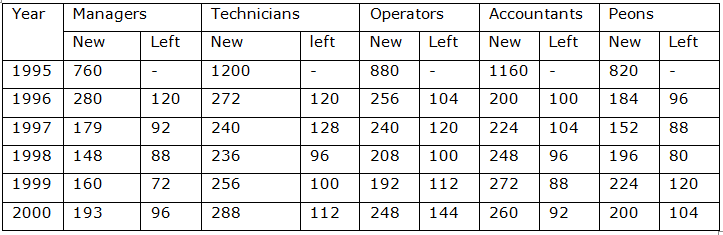6. What is the difference between the total number of Technicians added to the Company and the total number of Accountants added to the Company during the years 1996 to 2000?

(a) 128

(b) 112

(c) 96

(d) 88

(e) None of these

(d) 88 .

7. What was the total number of Peons working in the Company in the year 1999?

(a) 1312

(b) 1192

(c) 1088

(d) 968

(e) None of these

Required difference

= (272 + 240 + 236 + 256 + 288) – (200 + 224 + 248 + 272 + 260) = 88.

37. (b) Total number of Peons working in the Company in 1999

= (820 + 184 + 152 + 196 + 224) – (96 + 88 + 80 + 120)

= 1192

8. For which of the following categories the percentage increase in the number of employees working in the Company from 1995 to 2000 was the maximum?

(a) Mangers

(b) Technicians

(c) Operators

(d) Accountants

(e) None of these

(a) Mangers

Number of Managers working in the Company:

In 1995 = 760.

In 2000 = (760 + 280 + 179 + 148 + 160 + 193) – (120 + 92 + 88 + 72 + 96)

= 1252.

Percentage increase in the number of Managers

9. What is the pooled average of the total number of employees of all categories in the year 1997?

(a) 1325

(b) 1195

(c) 1265

(d) 1235

(e) None of these

(b) 1195

Total number of employees of various categories working in the Company in 1997 are:

10. During the period between 1995 and 2000, the total number of Operators who left the Company is what percent of total number of Operators who joined the Company?

(a) 19%

(b) 21%

(c) 27%

(d) 29%

(e) None of these

(d) 29%

[/su_spoiler

Directions (1-5): A school has four sections A, B, C, D of Class IX students.

The results of half yearly and annual examinations are shown in the table given below.

 Result No. of Students Sec- A Sec B Sec C Sec D Students failed in both Exams 28 23 17- 27 Students failed in half-yearly but passed in Annual Exams 14 12 8 13 Students passed in half-yearly but failed in Annual Exams 6 17 9 15 Students passed in both Exams 64 55 46 76

1. If the number of students passing an examination be considered a criteria for comparisons of difficulty level of two examinations, which of the following statements is true in this context?

(a) Half yearly examinations were more difficult.

(b) Annual examinations were more difficult.

(c) Both the examinations had almost the same difficulty level.

(d) The two examinations cannot be compared for difficulty level.

(e) None of these

(c) Both the examinations had almost the same difficulty level.

Number of students who passed half-yearly exams in the school

= (Number of students passed in half-yearly but failed in annual exams)

+ (Number of students passed in both exams)

= (6 + 17 + 9 + 15) + (64 + 55 + 46 + 76)

= 288.

Also, Number of students who passed annual exams in the school

= (Number of students failed in half-yearly but passed in annual exams)

+ (Number of students passed in both exams)

= (14 + 12 + 8 + 13) + (64 + 55 + 46 + 76)

= 288.

Since, the number of students passed in half-yearly = the number of students passed in annual exams. Therefore, it can be inferred that both the examinations had almost the same difficulty level.

Thus Statements (a), (b) and (d) are false and Statement (c) is true

2. How many students are there in Class IX in the school?

(a) 336

(b) 185

(c) 335

(d) 430

(e) None of these

(d) 430

Since the classification of the students on the basis of their results and sections form independent groups, so the total number of students in the class:

= (28 + 23 + 17 + 27 + 14 + 12 + 8 + 13 + 6 + 17 + 9 + 15 + 64 + 55 + 46 + 76)

= 430.

3. Which section has the maximum pass percentage in at least one of the two examinations?

(a) A section

(b) B section

(c) C section

(d) D section

(e) None of these

(d) D section

Pass percentages in at least one of the two examinations for different sections are: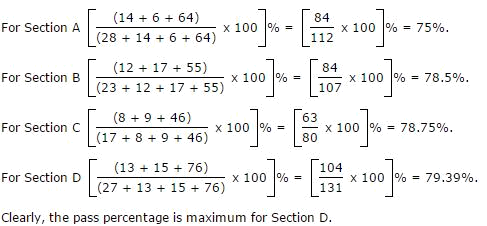4. Which section has the maximum success rate in annual examination?

(a) A section

(b) B section

(c) C section

(d) D section

(e) None of these

(a) A section

Total number of students passed in annual exams in a section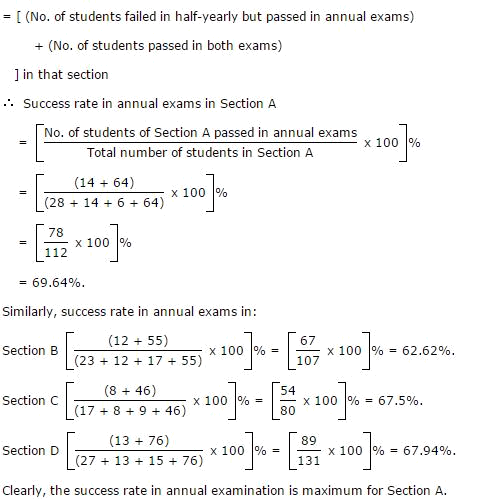5. Which section has the minimum failure rate in half yearly examination?

(a) A section

(b) B section

(c) C section

(d) D section

(e) None of these

(d) D section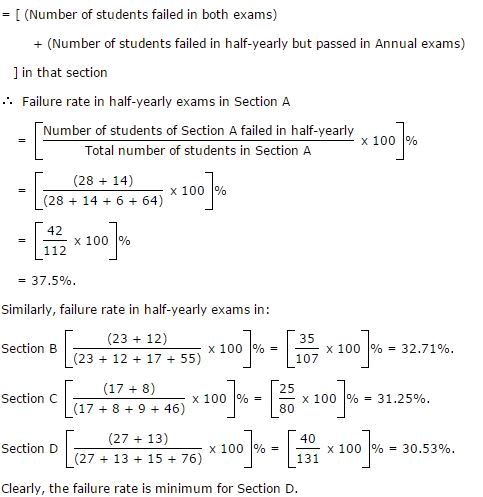Direction (6-10): The following table shows the number of new employees added to different categories of employees in a company and also the number of employees from these categories who left the company every year since the foundation of the Company in 1995.6. What is the difference between the total number of Technicians added to the Company and the total number of Accountants added to the Company during the years 1996 to 2000?

(a) 128

(b) 112

(c) 96

(d) 88

(e) None of these

(d) 88 .

7. What was the total number of Peons working in the Company in the year 1999?

(a) 1312

(b) 1192

(c) 1088

(d) 968

(e) None of these

Required difference

= (272 + 240 + 236 + 256 + 288) – (200 + 224 + 248 + 272 + 260) = 88.

37. (b) Total number of Peons working in the Company in 1999

= (820 + 184 + 152 + 196 + 224) – (96 + 88 + 80 + 120)

= 1192

8. For which of the following categories the percentage increase in the number of employees working in the Company from 1995 to 2000 was the maximum?

(a) Mangers

(b) Technicians

(c) Operators

(d) Accountants

(e) None of these

(a) Mangers

Number of Managers working in the Company:

In 1995 = 760.

In 2000 = (760 + 280 + 179 + 148 + 160 + 193) – (120 + 92 + 88 + 72 + 96)

= 1252.

Percentage increase in the number of Managers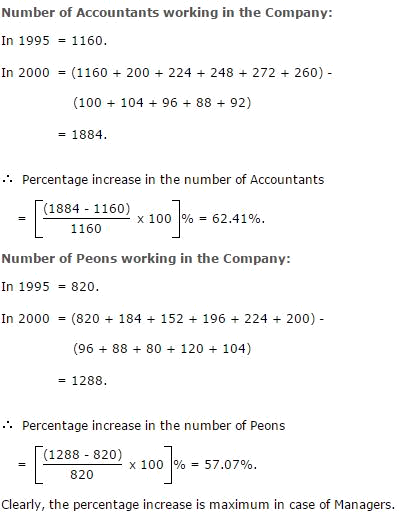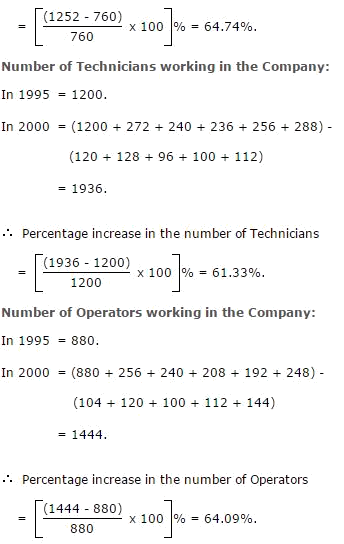9. What is the pooled average of the total number of employees of all categories in the year 1997?

(a) 1325

(b) 1195

(c) 1265

(d) 1235

(e) None of these

(b) 1195

Total number of employees of various categories working in the Company in 1997 are: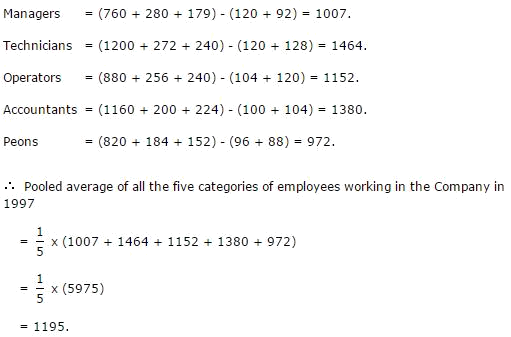10. During the period between 1995 and 2000, the total number of Operators who left the Company is what percent of total number of Operators who joined the Company?

(a) 19%

(b) 21%

(c) 27%

(d) 29%

(e) None of these